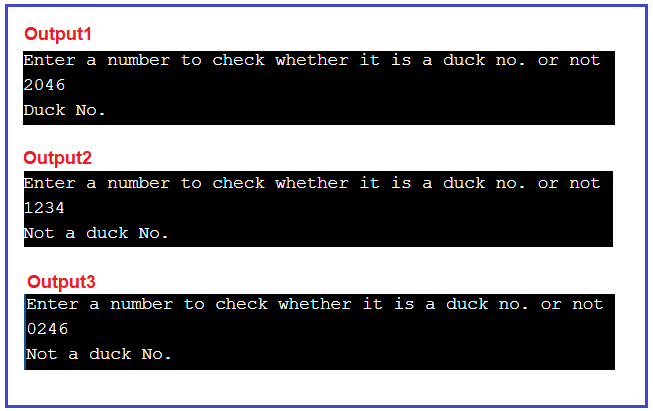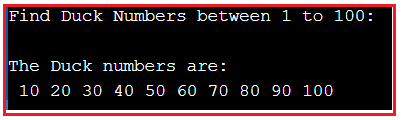# Duck Number in C#

## Duck Number in C# with Examples

In this article, I am going to discuss How to Implement the Duck Number Program in C# with Examples. Please read our previous article where we discussed How to Remove Duplicate Elements from an array in C# with two different approaches. Here, in this article, first, we will learn what is a Duck Number and then we will see how to implement the Duck Number program using the C# language.

##### What is a Duck Number?

A Duck number is a positive number that has zero (0) present in it. It may contain more than one 0 as an element but not as a leading element only. 0 should be present somewhere in the middle or as the last element of the digit. E.g.: 403,4002,43320,039830,034043 are all examples of duck number.

Condition: If a number has only led 0 as an element, then it will not be considered as a duck number. E.g.: 0432,0229,0643 are not examples of duck numbers.

##### How to Implement Duck Number in C#?

In this approach, we will convert integer numbers as string types and then extract each character one by one and check whether it equals ‘0’ or not.

1. Step1: convert entered value as a string.
2. Step2: Initialize a variable called ctr to store the value of the count of element ‘0’ in the string.
3. Step3: Using for loop we will take one by one character starting from index 1(we are not interested at index 0 because we duck number has 0’s in between or at last) and check for the ‘0’ element if it is equal to ‘0’ then simply increment the value of ctr.
##### Duck Number Program using C#
```using System;
public class CheckDuckNo
{
public static void Main (string[]args)
{
Console.WriteLine ("Enter a number to check whether it is a duck no. or not");
int number = Convert.ToInt32 (Console.ReadLine ());

String strNo = number.ToString ();

//lenght of string
int l = strNo.Length;

//to count available no. of zeros
int ctr = 0;

//To store the characters one by one in the string
char chr;

for (int i = 1; i < l; i++)
{
chr = strNo[i];
if(chr == '0')
{
ctr++;
break;
}
}

char f = strNo;

if (ctr > 0 && (f == '0' || f != '0'))
Console.Write ("Duck No.");
else
Console.Write ("Not a duck No.");
}
}
```
###### Output:Time Complexity: 0(n): As we are traversing the character array (string) only once.

##### How to find all the duck numbers between 1 to 100?

The following C# program shows how to find all the duck numbers between 1 to 100.

```using System;
public class CheckDuckNo
{
public static void Main (string[]args)
{
int dno, dkno, r, flg;
flg = 0;
Console.WriteLine ("Find Duck Numbers between 1 to 100: \n");
Console.WriteLine ("The Duck numbers are: ");

for (dkno = 1; dkno <= 100; dkno++)
{
dno = dkno;
flg = 0;
while(dno > 0)
{
if(dno % 10 == 0)
{
flg = 1;
break;
}
dno /= 10;
}
if(dkno > 0 && flg == 1)
{
Console.Write (" " + dkno);
}
}
}
}
```
###### Output:In the next article, I am going to discuss Disarium Number in C# with Examples. Here, in this article, I try to explain How to Implement the Duck Number Program in C# and I hope you enjoy this article.